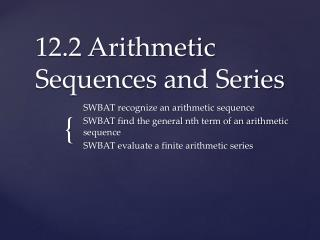Download Presentation12.2 Arithmetic Sequences and Series

# 12.2 Arithmetic Sequences and Series - PowerPoint PPT Presentation

12.2 Arithmetic Sequences and Series. SWBAT recognize an arithmetic sequence SWBAT find the general nth term of an arithmetic sequence SWBAT evaluate a finite arithmetic series. If each term in the sequence is found by adding a real number d to the previous term, so that. Arithmetic Sequences.I am the owner, or an agent authorized to act on behalf of the owner, of the copyrighted work described.
Download Presentation## 12.2 Arithmetic Sequences and Series

Download Policy: Content on the Website is provided to you AS IS for your information and personal use and may not be sold / licensed / shared on other websites without getting consent from its author.While downloading, if for some reason you are not able to download a presentation, the publisher may have deleted the file from their server.

- - - - - - - - - - - - - - - - - - - - - - - - - - E N D - - - - - - - - - - - - - - - - - - - - - - - - - -
Presentation Transcript
1. 12.2 Arithmetic Sequences and Series SWBAT recognize an arithmetic sequence SWBAT find the general nth term of an arithmetic sequence SWBAT evaluate a finite arithmetic series

2. If each term in the sequence is found by adding a real number d to the previous term, so that Arithmetic Sequences

3. Because , the number d Common Difference

4. Add 4 • Subtract 9 • Add ¾ • Subtract 5 • Add 2/3 Identifying the Common Difference in Arithmetic Sequences

5. for General (nth) Term of an Arithmetic Sequence

6. Find the 13th term of the arithmetic sequence of Finding the nth term of an arithmetic sequence

7. Find the 10th term of the arithmetic sequence 3, 10, 17, 24, … Finding the nth term of an arithmetic sequence

8. The 4th term of an arithmetic sequence is 16 and the 21st term is 67. Find and construct the sequence • Subtract 4 from 21 = 17 • 7, 10, 13, 16,… Finding the Arithmetic Sequence

9. Construct the arithmetic sequence whose 7th term is 26 and whose 13th term is 50 • Subtract 7 from 13 = 6 • 2,6,10,14,… Finding the Arithmetic Sequence